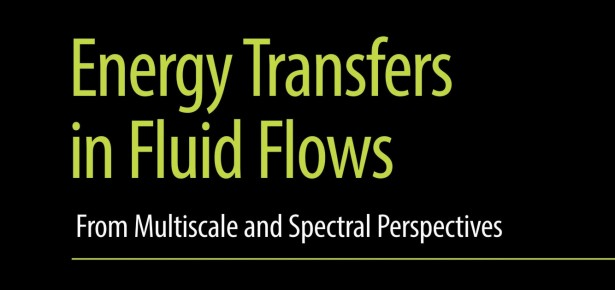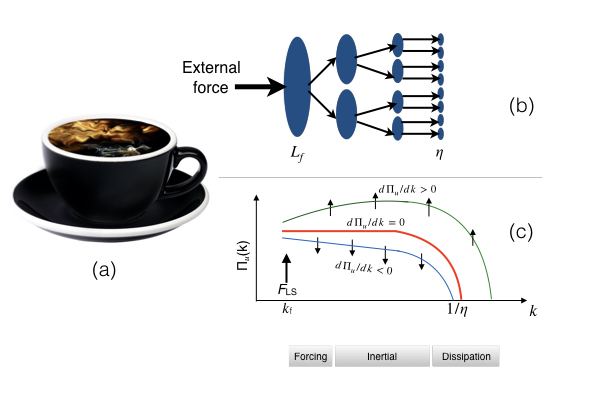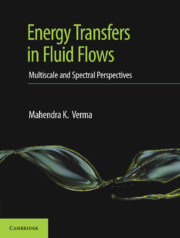Products and services

# Fifteen Eighty Four

9
Oct
2019

## Energy Transfers in Fluid FlowsUnderstanding turbulence is an important and challenging problem with a million dollar prize money on it.  We illustrate the complexity of a turbulent flow using an example.  Consider coffee being mixed vigorously, say by stirring (Figure 1(a)).  The kinetic energy fed at the large-scales cascades to intermediate scales and then to small scales, as shown in Figure 1(b).  This multiscale energy transfer process is described in great detail in my book Energy transfers in fluid flows.Figure 1: (a) Mixing of milk and coffee; (b) Cascading process in turbulence; (c) Energy flux Πu(k)

Let us understand the turbulent mixing of milk in coffee in some detail.  The stirring by spoon creates mini cyclone, technically called eddies, of the size of the cup (L).  This large eddy generates smaller eddies of size L/2, that in turn generates even smaller ones of size L/4, and so on (Figure. 1(b)).  This process continues till the smallest eddy of size η, where the fluid energy is converted to heat.

It is convenient and customary to compute the energy transfer from one scale to the next in Fourier space.   The wavenumbers are denoted by k, which is inverse of length; and the energy flux by Πu(k). In the coffee example, since there in no energy injection in the intermediate range, the same energy flux flows down the inertial range where viscous dissipation is weak.   Hence, Πu(k) = const., or dΠu(k)/dk =0 (see Figure 1(b,c)).  The corresponding energy spectrum is k-5/3.  This is Kolmogorov’s theory of turbulence.

Now imagine a strange experiment in which we mix polymers in coffee (not for drinking!).  Polymers are like small springs, and they extract a fraction of kinetic energy flux, Πu(k).  Hence Πu(k) decreases with k, or dΠu(k)/dk < 0.  On the other hand, if we heat up coffee, then the thermal energy enhances Πu(k), thus leading to dΠu(k)/dk > 0.  We illustrate these two cases in the Figure 1(c).   The above examples are illustrations of real-life flows—for example, Πu(k)/dk < 0 in magnetohydrodynamic turbulence and in flows with polymers.  But, Πu(k)/dk > 0 in thermal convection and in shear flows.  Thus, energy flux helps model these flows, as well as provide important inputs, e.g., turbulence drag reduction in polymeric flows and in magnetohydrodynamics.

In the monograph I describe basics of energy transfers and fluxes in turbulence, and then employ them to describe scaling and other properties of turbulence in hydrodynamics, magnetohydrodynamics, passive scale, buoyant flows, rotating flows, active scalars and vectors, compressible flows, etc.  Many of the above ideas have been discovered by our group, hence the book brings out a unique perspective on these topics.Cambridge University Press has produced an attractive book that,  I hope,  would be very useful to graduate students, scholars, and researchers.